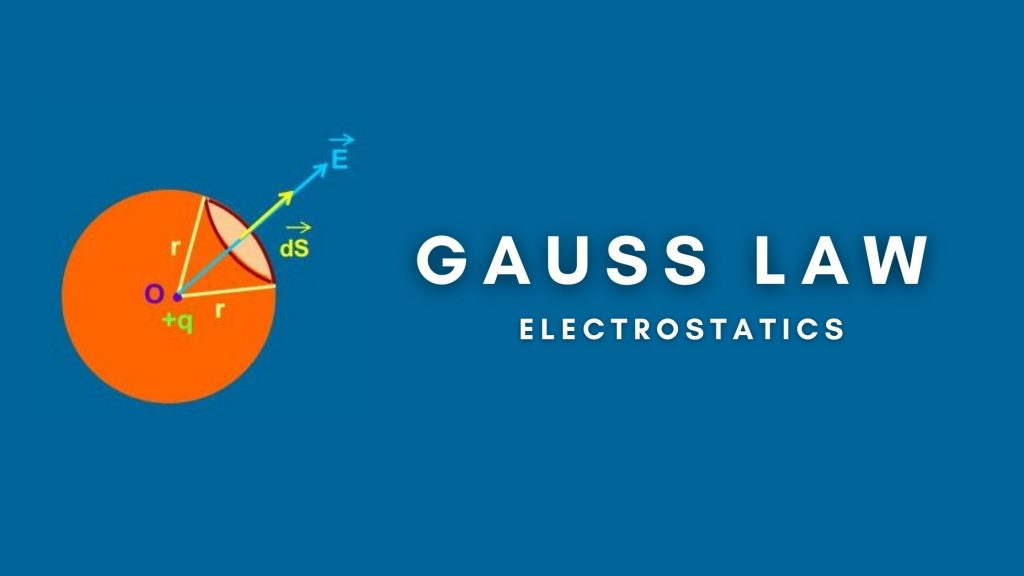Physics
Study Material

# Gauss Law in Electrostatics- Theorem, Formula, Applications

Gauss Law is like a shortcut to calculate flux from a closed surface. It has some limitations. By using certain logics we can overcome the limitations.

2 minutes long
Posted by Mahak Jain, 18/8/2021Hesap Oluştur

Got stuck on homework? Get your step-by-step solutions from real tutors in minutes! 24/7. Unlimited.

Gauss Law gives the measure of flux passing through a closed surface. Flux is measure of number of electric field lines passing across any surface. To read more about flux click here. This blog focuses specifically on Gauss Law. There is a limitation of Gauss Law that it can give flux linked to a closed surface only. Gauss Law is derived from Coulomb’s Law. So, if by any means Coulomb’s Law is proved false, Gauss Law will also be false and this blog will become useless.

## What is Gauss Law?

According to the Gauss law, the total flux linked with a closed surface is 1/ε0 times the charge enclosed by the closed surface.

Thus, ∮E.dA = q/ε0

## The Gauss Theorem

The net flux through a closed surface is directly proportional to the net charge in the volume enclosed by the closed surface. Net charge means algebraic sum of charges. For example if charges in a closed surface are +q, +3q, -q, -7q. So, the net charge is -4q.

Thus, φ = qnet0

Note that any charge outside the closed surface does not contribute to flux. Also flux passing through any surface is independent of shape of its shape.

## Gauss Law Formula and Derivation

Let us consider a sphere of radius r having charge q at its center. Consider a differential element as shown in figure.

dA vector is parallel to E vector. Thus, angle between them is 0°.

By definition, flux = φ = ∮E.dA

Since, every part of surface is equidistant from charge, E is constant.

Thus, φ = E ∫dA

Integration of dA will give the complete area of sphere.

Thus, ∫dA = 4πr2

Electric field at the surface of sphere is q/4πε0r2

Thus, φ = E.A

⇒ φ = q/4πε0r2 x 4πr2

φ = q/ε0